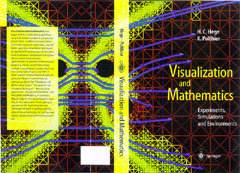Visualization and Mathematics I (Vismath 1995)Visualization and mathematics have begun a fruitful relationship, establishing links between problems and solutions of both fields. In some areas of mathematics, like differential geometry and numerical mathematics, visualization techniques are applied with great success. However, visualization methods heavily rely on mathematical concepts.

Applications of visualization in mathematical research and the use of mathematical methods in visualization have been topic of the international workshop VisMath 95 in Berlin. The workshop was met with great approval by mathematicians and computer graphic experts. It was considered a link between the fields "Visualization in Mathematics" and "Mathematical Methods in Computer Graphics".

This book contains a selection of contributions of this workshop which treat topics of particular interest in current research. Experts are reporting on their latest work, giving an overview on this fascinating new area. The reader will get insight to state-of-the-art techniques for solving visualization problems and mathematical questions.

We hope that the research articles of this book will stimulate the readers’ own work and will further strengthen the development of the field of Mathematical Visualization.

We thank T. Geßner, D. Kröner and M. Wierse for using their picture on the book cover. The picture shows two cylinders in a supersonic flow.

### Content

 Visualizing Mathematics Contributions by: K.A. Brakke, G. Chappell, G. Francis, C. Hartman, B. Hausmann, R.B. Kusner, G. Martin, R.J. Morris, D. Roseman, M. Ruhl, D. Saupe, H.-P. Seidel, B. Slopianka, I. Sterling and J.M. Sullivan. Geometric Algorithms and Experiments Contributions by: K.A. Brakke, K. Große-Brauckmann, D.H. Huson, B. Oberknapp, K. Polthier and J.M. Sullivan. Visualization Algorithms and Data Structures Contributions by: H. Battke, H.C. Hege, M. Kohler, H. Müller, M. Rumpf, B. Schupp and D. Stalling. Visualization Environments Contributions by: M. Alefeld, E. Beier, M. Dewar, D.W. Fellner, C. Gunn, J. Haber, A. Heim, J. Lemordant, A. Ortmann, U. Pinkall, K. Polthier, U. Schwarz, J. Walton. Visualization and Simulation Techniques Contributions by: R. Beck, P. Deuflhard, Th. Geßner, H.C. Hege, D. Kröner, R. Malladi, M. Seebaß, J.A. Sethian, D. Stalling and M. Wierse

### Keywords

Mathematical Visualization, Scientific Visualization, Numerical Mathematics, Scientific Computing, Computer Graphics

Mathematical Visualization, Scientific Visualization, Numerical Mathematics, Scientific Computing, Computer Graphics

1997, 386 pp., 230 figs., 43 figs. in color, hardcover
Springer Verlag Heidelberg. ISBN 3-540-61269-6.

Editor at Springer: Dr. Martin Peters